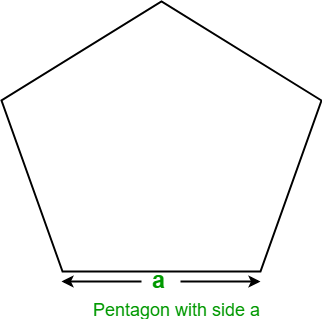GeeksforGeeks App
Open AppBrowser
Continue

# Program to find the Area of Pentagon

Given the side of a Pentagon, the task is to find the area of the Pentagon.
Examples:

Input : a = 5
Output: Area of Pentagon: 43.0119

Input : a = 10
Output: Area of Pentagon: 172.047745

A regular pentagon is a five sided geometric shape whose all sides and angles are equal. Its interior angles are of 108 degrees each and its exterior angles are 72 degrees each. The sum of interior angles of a pentagon is 540 degrees.Let a be the side of Pentagon, then the formula to find the area of Pentagon given by
Area =## C++

 // C++ program to find the area of Pentagon#includeusing namespace std; // Function to find area of pentagonfloat findArea(float a){    float area;       // Formula to find area    area = (sqrt(5 * (5 + 2 * (sqrt(5)))) * a * a) / 4;       return area;} // Driver codeint main(){    float a = 5;         // function calling    cout << "Area of Pentagon: " << findArea(a);         return 0;}

## Java

 // Java program to find the area of Pentagonimport java.io.*; class GFG {         // Function to find area of pentagon    static float findArea(float a)    {        float area;               // Formula to find area        area = (float)(Math.sqrt(5 * (5 + 2                * (Math.sqrt(5)))) * a * a) / 4;               return area;    }         // Driver code    public static void main (String[] args)    {        float a = 5;                 System.out.println("Area of Pentagon: "                               + findArea(a));    }}

## Python3

 # Python3 program to find# the area of Pentagon # Import Math module# to use sqrt functionfrom math import sqrt # Function to find# area of pentagon def findArea(a):         # Formula to find area    area = (sqrt(5 * (5 + 2 *           (sqrt(5)))) * a * a) / 4    return area      # Driver codea = 5 # call function findArea()# to calculate area of pentagon# and print the calculated areaprint("Area of Pentagon: ",               findArea(a))  # This code is contributed# by ihritik

## C#

 // C# program to find// the area of Pentagonusing System; class GFG{         // Function to find    // area of pentagon    static float findArea(float a)    {        float area;             // Formula to find area        area = (float)(Math.Sqrt(5 * (5 + 2 *                      (Math.Sqrt(5)))) *                             a * a) / 4;             return area;    }         // Driver code    public static void Main ()    {        float a = 5;                 Console.WriteLine("Area of Pentagon: "+                                  findArea(a));    }} // This code is contributed// by anuj_67.

## PHP

 

## Javascript

 

Output:

Area of Pentagon: 43.0119

Time complexity: O(1)

Auxiliary Space: O(1), since no extra space has been taken.

My Personal Notes arrow_drop_up